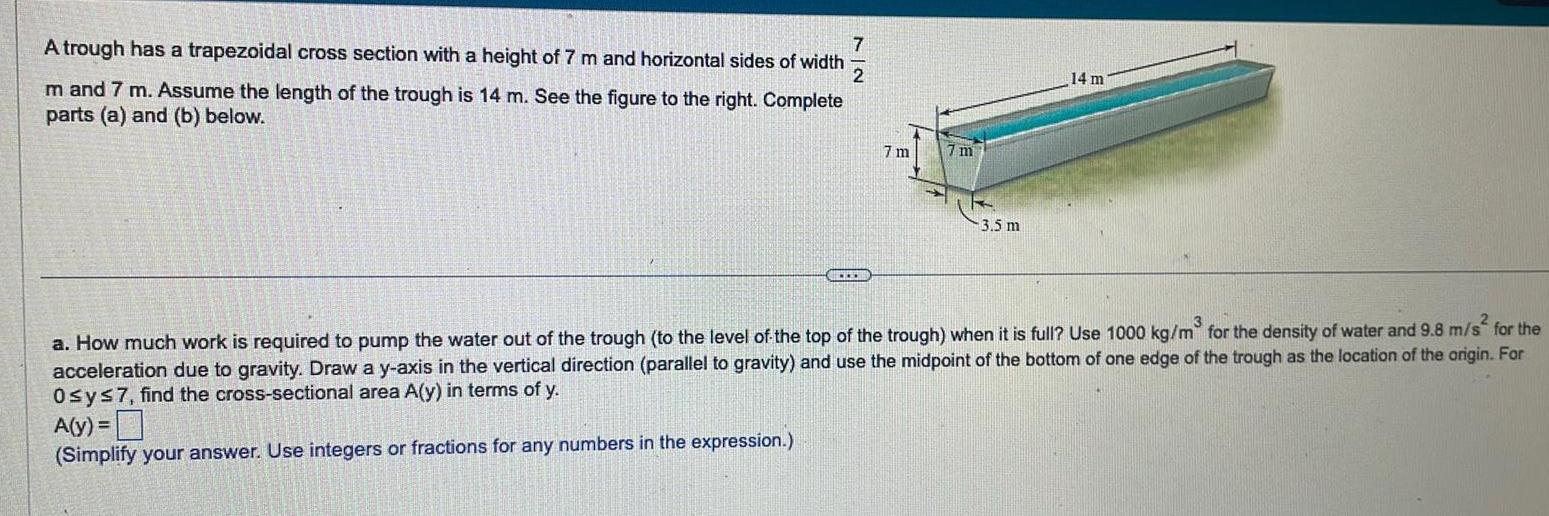Question:

# A trough has a trapezoidal cross section with a height of 7

Last updated: 11/19/2023A trough has a trapezoidal cross section with a height of 7 m and horizontal sides of width 7 2 m and 7 m Assume the length of the trough is 14 m See the figure to the right Complete parts a and b below MICKERS 7 m 7 m 3 5 m 14 m a How much work is required to pump the water out of the trough to the level of the top of the trough when it is full Use 1000 kg m for the density of water and 9 8 m s for the acceleration due to gravity Draw a y axis in the vertical direction parallel to gravity and use the midpoint of the bottom of one edge of the trough as the location of the origin For Osy 7 find the cross sectional area A y in terms of y A y Simplify your answer Use integers or fractions for any numbers in the expression